# How Code 19 can protect all the letters of the Quran

..

I have read articles from Rashad, submission.org, masjidtucson.org, and others. But a question remains in my head about how this code number 19 can also protect all the letters of the Quran.

Since they don’t share the text of the Quran for download, I tried to verify it myself using Buckwalter data from corpus.quran.com, then patched it using the following references from submission.org:

I assume all readers already know what Arabic gematria is. But if you don’t know it, you can read it here or here first. In essence, each letter in Arabic has its own value, as can be seen in the table below:

For example, in the Quran there are several chapters with the initials Alif, Lam, Mim, then its gematrical values is: Alif = 1, Lam = 30, Mim = 40, so 1 + 30 + 40 = 71. And in this article the gematrical value of each letter will be shortened to Gv.

And this is what Rashad did not explain in his articles, about how this code number 19 can protect not only initial letters, but also all un-initial’s letters in the Quran. And after I calculated (including Basmalah in every chapter except for chapter 1 and 9), the result was: 3 2 8 1 6 0 letters.

Let’s check out this result with this code number 19, and see how awesome it is :)

..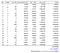Table. 3 Code number 19’s pattern based on the Basmalah letters.

Here we can see the letters that make up this Basmalah can also act as Guardian or Quick Tester, and if the result of its calculation is not divisible by 19, then there may be a writing/printing error or something else.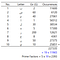Table 4. Code number 19’s pattern based on unique Basmalah letters.

Again, Basmalah acts as Guardian or Quick Tester, if the result of its calculation is not divisible by 19, then there may be a writing/printing error or something else. And interesting to note that Basmalah is composed of 19 letters with 10 unique letters, and in the Mathematics 19 Base 19 = 10

..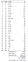Table. 5 Code 19’s pattern based on all Initials Letters of the Quran.

Here we can see that one of the functions of Gv is to maintain the value/quality of the total number/quantity of Quran letters. Because it could be that in the practice of its writing or calculating, the numbers are the same (328160), but for example, there is one letter that should be written Ta (ت) instead written as Tha (ث) which of course will affect the total value of its Gv, which, if added up will certainly not be divisible by 19 which indicates a writing error or something else.

And it is interesting to note what our French brother found that if the total Gv of these letters is subtracted with the total Gv of the un-numbered verses (112 Basmalah), then the result will be divisible by 19.
23463124 -(112 x 786) = 23375092 = 19 x 1230268.
And its prime factor = 2 x 2 x 13 x 19 x 59 x 401.

..

In order to maintain initialed chapters (whether it is an initial letter or not), with this code number 19 was found at least seven (7) pairs of chapters with the following patterns:

Each of pairs of these chapters can be seen in the tables below, and from here we will know the function of the initial letters and their Gv.

As can be seen in the table above, in the Quran, there are six (6) chapters with the initials A.L.M, which are: the 2nd, 3rd, 29th, 30th, 31st and 32nd chapter.
Sum of A.L.M of these six (6) chapters is divisible by 19. In order to make — sum of other letters than A.L.M in these six (6) chapters — are divisible by 19, then it must be added with the Gv of this A.L.M (71).
And the pattern like this also can be applied to other Initialed chapters in the Quran, but with different pairs according to its key letter.

..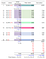Table. 7 Pair #2 Protecting ALMS, ALR, ALMR, KHY’AS, Q and N

..

This is one very impressive pattern. To protect initial letters T.H; T.S.M; and T.S; it takes the letter M in the next four (4) chapters which are incidentally having initials A.L.M. Because what are calculated in the column “Initials Occurrences” in chapters 29,30,31 and 32 is only letter M, so what is added to protect the other un-initial letters is only the Gv of the letter M, which is 40.

..

The pattern above is the same as the previous pattern, but here, what are calculated in the column “Initials Occurrences” for chapters 19 and 7 is only the letter S alone, so only the Gv of the letter S alone which is added, which is 90.

..

From the table above it can be seen that chapters with the initials H.M can form three (3) patterns in which the total number of its initial letters are divisible by 19.

..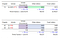Table. 11 Pair #6 and #7. These two pairs are special, they don’t need its Gv to protect its letters.

These two pairs of chapters do not need their Gv to protect all of the letters in their chapters, in fact, these two pairs help the other chapters to protect their letters.

..Table. 12 Pattern based on the Initial Chapters of the Quran and its total letters.

..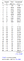Table 13. Pattern based on Un-initial Chapters of the Quran and its total letters.

..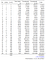Table 14. Pattern based on total Quran letters and its distribution.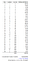Table 15. Pattern based on total Quran letters and its distribution.

..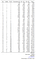Table 16. Pattern based on all letters but without all initial letter in its chapter.

..

With many and varied patterns of code number 19 in the Quran, and assisted by advances in science, especially computerization, it will be very easy for us to detect errors in its writing or anything else (e.g.: forgery attempts). With the results of this test, we can conclude:

• The Quran must be written/printed with Uthmani Rasm like Medina Mushaf, and this is different from the Quran in many non-Arabic speaking countries which are mostly written/printed with Imlai/Indopak Rasm.
• Basmalah in each chapter (except chapter 1 and 9) must be combined with other verses and its verse number is zero (0), and not written/printed separately from other verses.
• There are at least twenty-five (25) typographical errors in the Medina Mushaf (Table. 1)
• The Quran consists of 114 (19x6) chapters and 6346 (19x334) verses, and should be written/printed without 9:128–129.

Are All patterns of code number 19 above just a coincidence? If you are interested in proving it yourself, you can download the spreadsheet data (v2021–03–03) here: clickme
And to download the text of the Quran (pdf file) that has been mathematically verified by the code number 19, you can download it here: clickme

Finally, this article was written and published to Medium on Friday, February 19, 2021, or 19022021 or 19 x 101159

Coincidence?

Human being